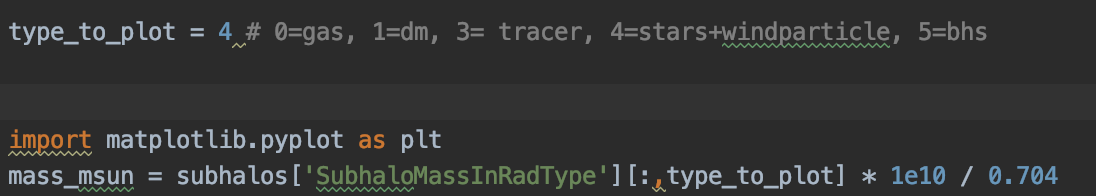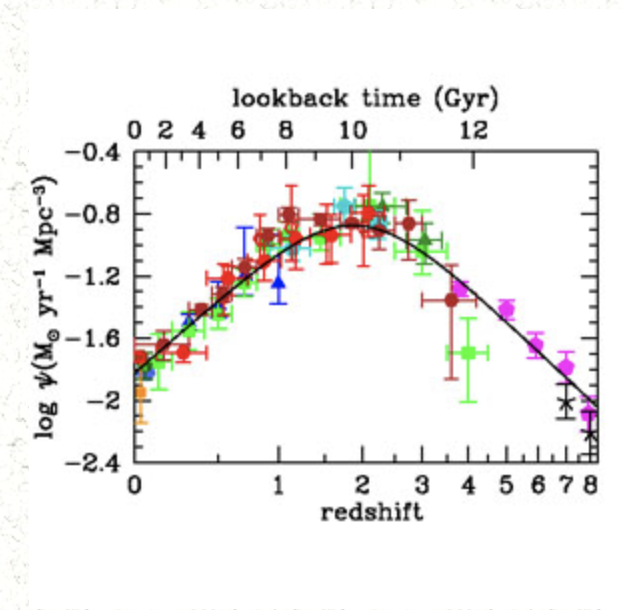### Questions for Illustris simulation data and galaxy main sequenceYunwoo Nam
• 26 Nov '19

I'm a student with a major in astronomy in college in Korea

First. I want to plot galaxy main sequence according to zthis code is alright? I want to use stellar mass. so, I think
but this code is dimension error. why?

Second I want to plot starformation density rate according to z
I think each galaxy main sequence plot data is list for.m
And My plane is sum all star formation rate according to z
and plotting starformation density rate

how to sum of starformaiton rate density. this data is list form? and how can I handle it

for exampleSorry for my poor English and Thank youDylan Nelson
• 26 Nov '19

Hi Yunwoo,

Your code for `mass_msun` seems ok, what is the error? What is `subhalos` (how do you load it)?

For SFRD(z), you could load `SubhaloSFR` (one number per galaxy) at one snapshot, and sum this numpy array. Then loop over all snapshots, e.g.

``````sfrd = []
snaps = np.arange(10,100)

for snap in snaps:
sfrd.append( sfr_galaxies.sum() )

ax.plot( snaps, sfrd )
``````Yunwoo Nam
• 26 Nov '19

my new mass code : mass_msun = subhalos['SubhaloMassInRadType'][:,4] * 1e10 / 0.704

first
I understand ['SubhaloMassInRadType'] is STARS & WIND PARTICLES
If ['SubhaloMassInRadType'] is stellar mass, multiply 1e10 / 0.704 should not be multiplied.

second
How can I all snapshots 135 ~ 0 at once ??Dylan Nelson
• 26 Nov '19

Hello,

`SubhaloMassInRadType` is a mass of only stars, it does not contain wind.

It requires a unit conversion to obtain solar masses, so you must multiply by (1e10/0.704).Yunwoo Nam
• 27 Nov '19

I downloaded only Illustris-3, FoF & Subfind group cat file( z 135 ~ 10, step 10 ). My notebook's memory is not enough to save snapshot files and
I will plane to use FoF & Subfind group cat. Is it hard to get to SFRD(z) only use FoF & Subfind group cat?

I think each 'SubhaloMassInRadType' is list data so I sum 'SubhaloMassInRadType' data
This value (Sum of starformation rate) is not SFRD? because we know zDylan Nelson
• 29 Nov '19

Hello,

There are many possible definitions of SFRD. If you sum from the FoF+Subfind catalog, then you will obtain a SFRD which includes SFR within those objects.

If you sub-select a fraction of the FoF+Subfind catalog, then you will obtain a SFRD which includes, similarly, only the SFR within that subset of objects.

The units of `SubhaloSFR` are solar masses per year. If you divide by the volume, you obtain a SFRD value.

• Page 1 of 1